# Electric Flux And Field Due To Charge Distribution MCQ

## 10 Questions MCQ Test Electricity & Magnetism | Electric Flux And Field Due To Charge Distribution MCQ

Description
Attempt Electric Flux And Field Due To Charge Distribution MCQ | 10 questions in 45 minutes | Mock test for IIT JAM preparation | Free important questions MCQ to study Electricity & Magnetism for IIT JAM Exam | Download free PDF with solutions
QUESTION: 1

### Two concentric sphere of radii R and r have similar charges with equal surface charge density σ. The electric potential at their common centre is

Solution:

By superposition Principle.
Electric Potential at common centre is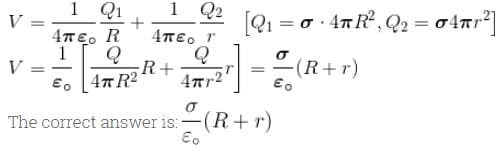QUESTION: 2

### A long string with a charge of λ per unit length passes through an imaginary cube of edge l. The maximum possible flux of electric field through the cube will be

Solution:

Maximum length of string =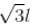Maximum enclosed charge =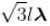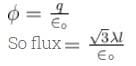The correct answer is: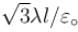QUESTION: 3

### Force of attraction between the plate of a parallel plate capacitor is

Solution: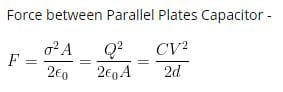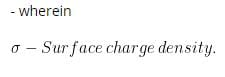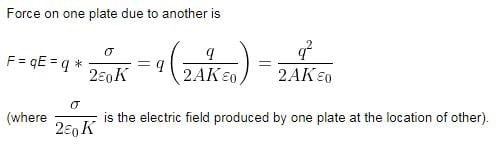QUESTION: 4

Consider two concentric conducting spherical shell with inner and outer radii abc and d as shown in the figure. Both the shell are given Q amount of positive charges. The electric field in different regions are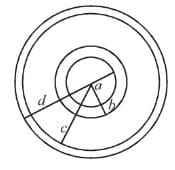Solution:

For r < a, applying Gauss’s law.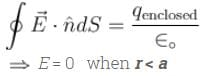∴ q = 0
Also since shells are conducting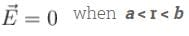For b < r <  by Gauss’s law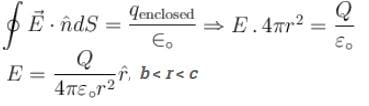For r > d, applying Gauss’s law, we get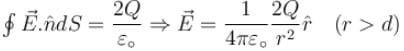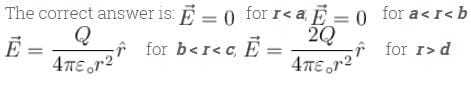QUESTION: 5

Electric field in the region is given by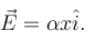Here, α is a constant of proper dimensions. The total flux passing through a cube bounded by the surfaces x = l , x = 2l, y = 0, y = l, z = 0, z = l.

Solution:

Except the faces ABCD and EFGH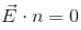for all the faces as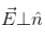So, flux through the cube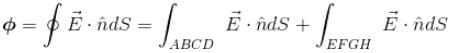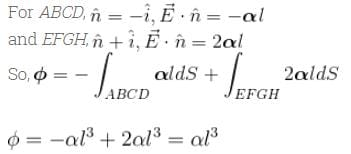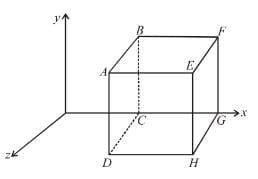QUESTION: 6

A spherical condenser has inner and outer sphere of radii a  and b respectively. The space between the two is filled with air. The difference between the capacities of two condensers formed when outer sphere is earthed and when inner sphere is earthed will be

Solution:

Capacity of spherical condenser when outer sphere is earthed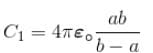Capacity of spherical condenser when inner sphere is earthed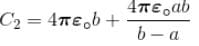Difference in their capacity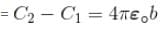The correct answer is: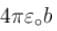QUESTION: 7

A diagram of an irregularly shaped charged conductor is shown at the right figure. Four location along the surface are labeled ABCD. Rank these locations in increasing order of the strength of their electric field beginning with the smallest electric field.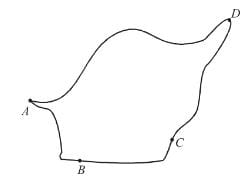Solution:

For conducting surface, electric field are strongest at the location where the curvature is the greatest. A projection (such as at point A) is the extreme case of high carvature. A flat section of a surface (such as point B) is on the opposite extreme with the no curvature. So, point B is listed first and point A is listed last. Since point D is on a section of the surface which curve more than point C. The field at point C is more than field at point B.

The correct answer is: B < C < D < A

QUESTION: 8

A positively charge non-conducting disc having uniform surface change density α coulomb/m2. The difference in the potential at its centre and at its edge is given by.

Solution:

Potential at the centre of disc. By taking a element of charge in the form of ring of radius r and thickness dr.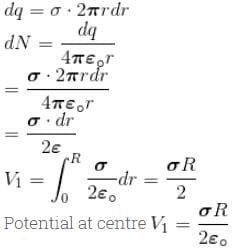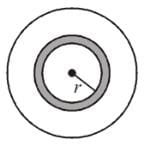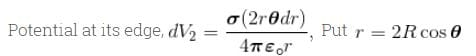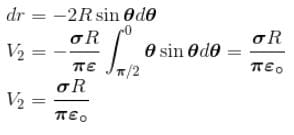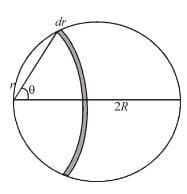Now difference between the potential at the centre and at its edge is given by
V = V1 – V2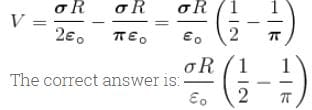QUESTION: 9

A metallic sphere of radius r and carrying a charge q is enclosed by a dielectric shell of thickness δ outer radius r2 and relative permittivity ε The medium elsewhere is air. The potential V(r) for r > r2 is

Solution:

E = P = D = 0  [Inside the metal sphere]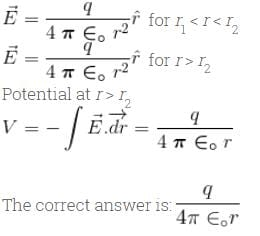QUESTION: 10

A charged ball B hangs from a silk thread S, which make an angle θ and a large conducting sheet P as shown in the figure. The surface charge density σ of the sheet is proportional to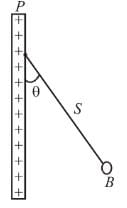Solution:

For equilibrium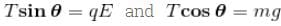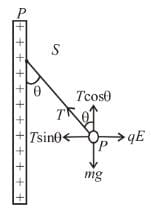Dividing, we get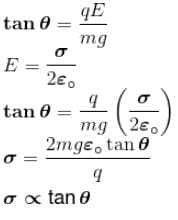The correct answer is: tan θUse Code STAYHOME200 and get INR 200 additional OFF Use Coupon Code